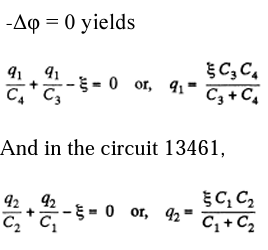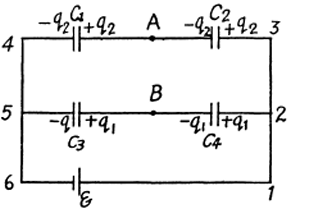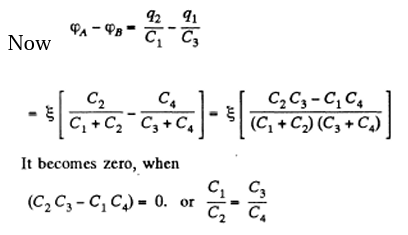# I.E. Irodov Solutions on Electric Capacitance Energy of an Electric Field

Electric Capacitance Energy of an Electric Field is an important chapter in Physics that mainly deals with the electric field and properties with capacitors. The chapter comprises various numerical based on the syllabus and also the practical applications. I.E. Irodov Physics provides the solutions to different varieties of numerical. Electric Capacitance Energy of an Electric Field is a necessary resource element that is needed by all students to score good marks in Class 12 Physics examinations and entrance examinations. It is one of the best ways to study and practice for physics examinations to score high. The problems asked here are mainly based on electric field, current, speed, voltage and so on.

The problems and questions in this chapter mainly comprise capacitors connected in series, parallel, change in energy when electric field is applied or removed. The concept explained here is crystal clear and goes hand to hand with the syllabus. These problems connect the theoretical concepts with practical and also enhance their applications in daily life and activities. Students aiming for a high score in competitive examination must solve all the given problems for easy understanding and to have a grip over the syllabus and concept.

One can get access to all sorts of problems based on each and every formula, equations and method. Solving these types of problems builds the confidence of students and enables them to have a grip over the subject.

### I.E. Irodov Solutions on Electric Capacitance Energy of an Electric Field

1. Find the capacitance of an isolated ball R shaped conductor of radius surrounded by an adjacent concentric layer of dielectric with permittivity ε and 1 outside radius R2.

Solution:

1. Let us mentally impart a charge q on the conductor, then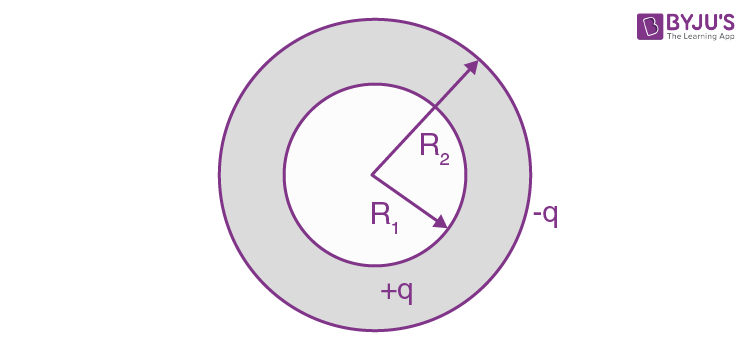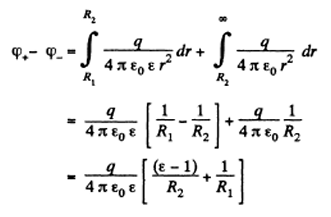Hence the sought capacitance is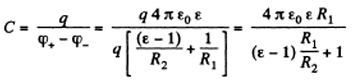2. Two parallel plate air capacitors, each of capacitance C, were connected in series to a battery with EMF E. Then one of the capacitors was filled up with uniform dielectric with permittivity ε. How many times did the electric field strength in that capacitor decrease? What amount of charge flows through the battery?

Solution:

1. From the symmetry of the problem, the voltage across each capacitor is

$\Delta \phi =\frac{\varepsilon }{2}$ and charge on each capacitor is

$q=\frac{C\varepsilon }{2}$

in the absence of dielectric Now when the dielectric is filled up in one of the capacitors, the equivalent capacitance of the system,

$C'_{0}=\frac{C_{\varepsilon }}{1+\varepsilon }$

And the potential difference across the capacitor, which is filled with dielectric

$\Delta \phi '=\frac{q'}{\varepsilon C}=\frac{C_{\varepsilon }}{1+\varepsilon }. \frac{E}{C_{\varepsilon }}=\frac{E}{1+\varepsilon }$

But φ α E

So, as φ decreases $\frac{1}{2}(1+\varepsilon )$ times the field strength, also decreases by the same factor and flow of charge $\Delta q=q'-q$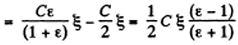3. The space between the plates of a parallel-plate capacitor is filled consecutively with two dielectric layers 1 and 2 having the thicknesses d1 and d2 and the permittivity’s ε1and ε2 respectively. The area of each plate is equal to S. Find:

1. (a) the capacitance of the capacitor;

2. (b) the density σ' of the bound charges on the boundary plane if the voltage across the capacitor equals V and the electric field is directed from layer 1 to layer 2.

Solution:

1. (a) As it is the series combination of two capacitors,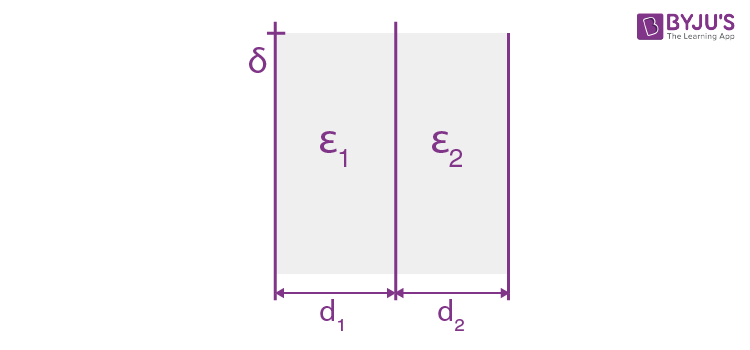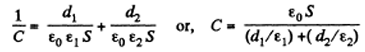(b) Let, a be the initial surface charge density, the density of bound charge on the boundary plane.4. The gap between the plates of a parallel-plate capacitor is filled with isotropic dielectric whose permittivity ε varies linearly from ε1 to ε22 > ε1) in the direction perpendicular to the plates. The area of each plate equals S, the separation between the plates is equal to d. Find:

1. (a) the capacitance of the capacitor;

2. (b) the space density of the bound charges as a function of ε if the charge of the capacitor is q and the field E in it is directed toward the growing ε values.

Solution:

1. (a) We point the jt-axis towards the right and place the origin on the left-hand side plate. The left plate is assumed to be positively charged. Since e varies linearly, we can write,

ε(x) = a + bx

where a and b can be determined from the boundary condition. We have

ε=ε1 at x=0 and ε=ε2 at x=d

Thus

$\varepsilon (x)=\varepsilon _{1}+\left ( \frac{\varepsilon _{2}- \varepsilon _{1}}{d} \right )x$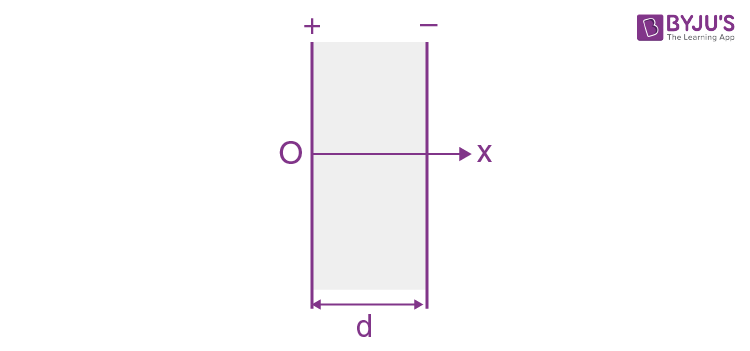Now potential difference between the plates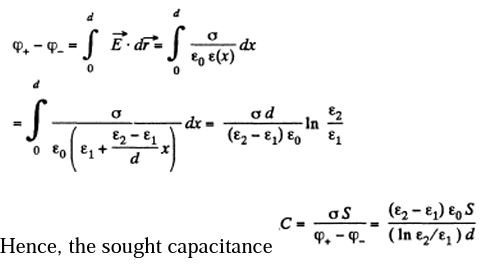(b) $D=\frac{q}{s}$ and $P=\frac{q}{s}-\frac{q}{S\varepsilon (x)}$

And the space density of bound charges is

$P'=- div p= -\frac{q(\varepsilon _{2}-(\varepsilon _{1})}{Sd\varepsilon ^{2}(x)}$

5. Find the capacitance of a spherical capacitor whose electrodes have radii R1 and R2 > R1 and which is filled with isotropic dielectric whose permittivity varies as ε = a/r, where a is a constant, and r is the distance from the centre of the capacitor.

Solution:

1. Let, us mentally impart a charge q to the conductor. Now potential difference between the plates,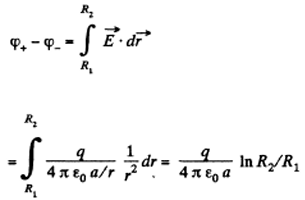Hence the sought capacitance,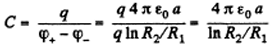6. A cylindrical capacitor is filled with two cylindrical layers of dielectric with permittivity’s ε1 and ε2. The inside radii of the layers are equal to R1 and R2 > R1. The maximum permissible values of electric field strength are equal to E1m and E2m for these dielectrics. At what relationship between ε, R, and Em, will the voltage increase result in the field strength reaching the breakdown value for both dielectrics simultaneously?

Solution:

1. Let λ be the linear charge density then,

$E_{1m}=\frac{\lambda }{2\pi \varepsilon _{o}R_{1}\varepsilon _{1}}$---(1)

$E_{2m}=\frac{\lambda }{2\pi \varepsilon _{o}R_{2}\varepsilon _{2}}$---(2)

The breakdown in either case will occur at the smaller value of r for a simultaneous breakdown of both dielectrics.

From (1) and (2)

E1mR1ε1=E2mR2ε2

7. There is a double-layer cylindrical capacitor whose parameters are shown in Figure. The breakdown field strength values for these dielectrics are equal to E1 and E2 respectively. What is the breakdown voltage of this capacitor if ε1R1E1< ε2R2E2?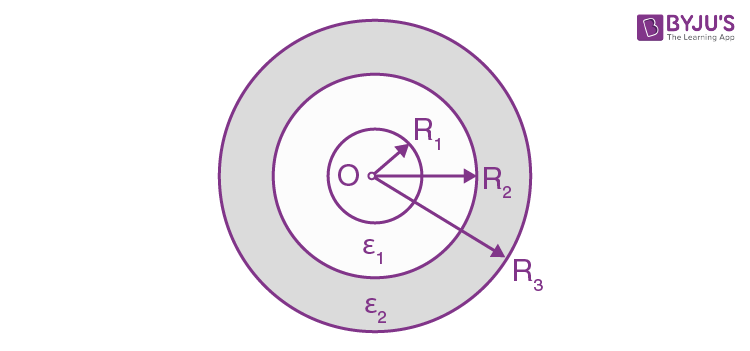Solution:

1. Let, λ be the linear charge density then, the sought potential difference,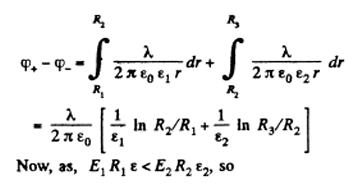$\frac{\lambda }{2\pi \varepsilon _{o}}=E_{1}R_{1}\varepsilon _{1}$

is the maximum acceptable value, and for values greater than ε1R1E1 dielectric breakdown will take place, Hence, the maximum potential difference between the plates,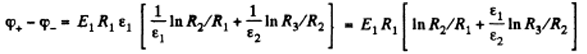8. Two long straight wires with equal cross dielectric sectional radii are located parallel to each other in the air. The distance between their axes equals b. Find the mutual capacitance of the wires per unit length under the condition b linear ≫ a.

Solution:

1. Let us suppose that the linear charge density of the wires be λ then, the potential difference

$\phi _{2}-\phi _{1}=\phi -(-\phi )=2\phi$

The intensity of the electric field created by one of the wires at a distance x from its axis can be easily found with the help of the

$E=\frac{\lambda }{2\pi \varepsilon _{0}x}$

Then

$\phi =\int_{a}^{b-a}E dx= \frac{\lambda }{2\pi \varepsilon _{0}}\ln \frac{b-a}{a}$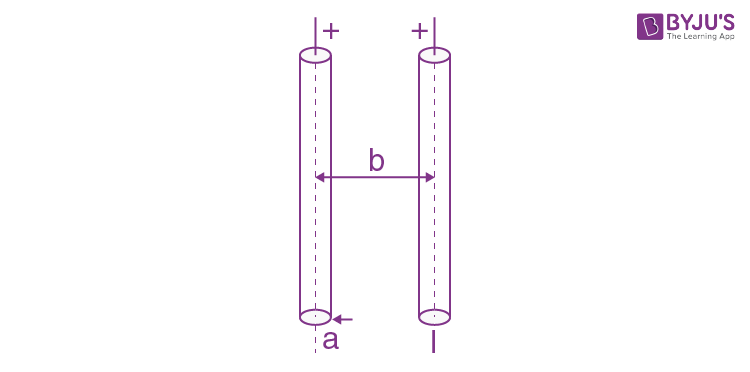Hence,capacitance,per unit length,

$\frac{\lambda }{\phi _{+}-\phi _{-}}=\frac{2\pi \varepsilon _{0}}{\ln \frac{b}{a}}$

9. A long straight wire is located parallel to an infinite conducting plate. The wire cross-sectional radius is equal to a, the distance between the axis of the wire and the plane equals b. Find the mutual capacitance of this system per unit length of the wire under the condition a≪b.

Solution:

1. The field in the region between the conducting plane and the wire can be obtained by using an oppositely charged wire as an image on the other side.Then the potential difference between the wire and the plane,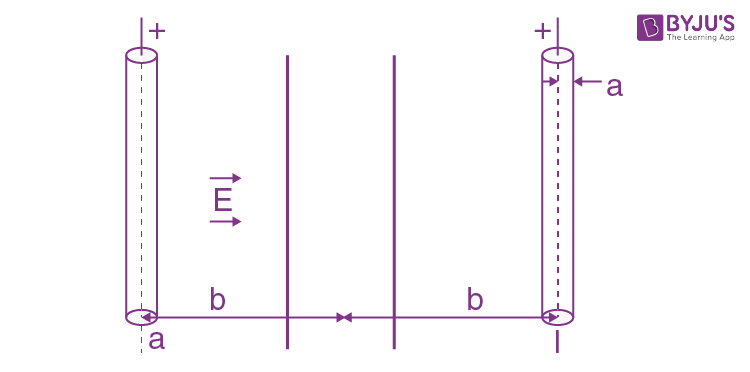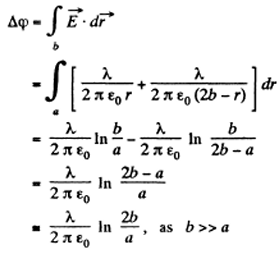Hence the sought capacitance, the system per unit length of the wire is

$\frac{\lambda }{\Delta \phi }=\frac{2\pi \varepsilon _{0}}{\ln \frac{2b}{a}}$

10. Find the capacitance of a system of two identical metal balls of radius a if the distance between their centres is equal to b, with b in a uniform dielectric with permittivity a.

Solution:

1. When b >> a, the charge distribution on each spherical conductor is practically unaffected by the presence of the other conductor. Then, the potential φ + (φ) on the positive (respectively negative) charged conductor is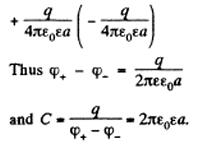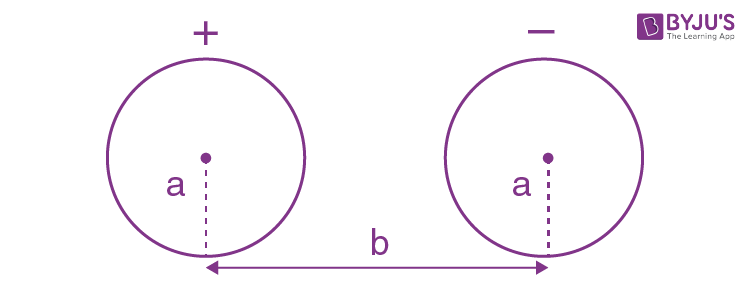Note: if we require terms which depend on a/b, of charge on the conductors. we have to take account of distribution on the charge of conductors.

11. Determine the capacitance of a system consisting of a metal ball of radius a and an infinite conducting plane separated from the centre of the ball by the distance 1 if l ≫ a.

Solution:

1. We will apply the method of image. Then the potential difference between the positively charged sphere and the conducting plane is one half the nominal potential difference between the sphere and its image

$\Delta \phi =\frac{1}{2}(\phi _{+}-\phi _{-})=\frac{q}{4\pi \varepsilon _{0}a}$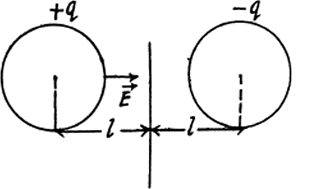Thus

$C=\frac{q}{\Delta \phi }=4\pi \varepsilon _{o}a$ for l>>a

12. Find the capacitance of a system of identical capacitors between points A and B as shown in the figure.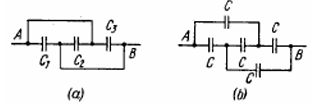Solution:

1.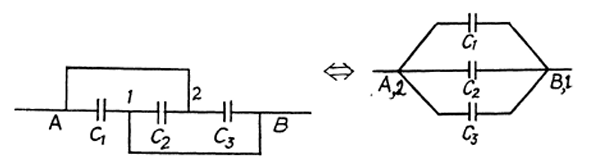(a)ϕ1B and ϕ2A

The arrangement of capacitors shown in the problem is equivalent to the arrangement shown in the figure below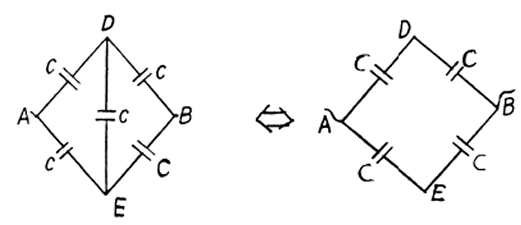And hence the capacitance between A and B is,

C=C1+C2+C3

(b) From the symmetry of the problem, there is no Potential. difference between D and E. So, the combination reduces to a simple arrangement shown in the Figure and hence the net capacitance,

C0=C/2+C/2+C

13. Four identical metal plates are located in air at equal distances d from one another. The area of each plate is equal to S. Find the capacitance of the system between points A and B if the plates are interconnected as shown in figure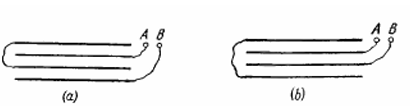Solution:

1. In the given arrangement, we have three capacitors of equal capacitance,$C=\frac{\varepsilon _{o}S}{d}$ and the first and third plates are at the same potential.

Hence, we can resolve the network into a simple form using series and parallel grouping of capacitors, as shown in the figure. Thus the equivalent capacitance

$C_{0}=\frac{(C+C)C}{(C+C)+C}=\frac{2}{3}C$

(b) Let us mentally impart the charges +q and q to plates 1 and 2 and then distribute them to other plates using charge conservation and electric induction. As the potential difference between the plates 1 and 2 is zero,

$-\frac{q_{1}}{C}+\frac{q_{2}}{C}-\frac{q_{1}}{C}=0$ where $C=\frac{\varepsilon _{o}S}{d}$

Or q2=2q1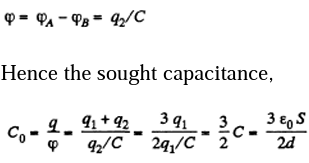14. A capacitor of capacitance C1 = 1.0 μ.F withstands the maximum voltage V1 = 6.0 kV while a capacitor of capacitance C2 = 2.0 μF, the maximum voltage V2 = 4.0 kV. What voltage will the system of these two capacitors withstand if they are connected in series?

Solution:

1. Amount of charge, that the capacitor of capacitance C1 can withstand, q1 = C1 V1 and similarly the charge, that the capacitor of capacitance C2 can withstand, q2 = C2 V2. But in a series combination, the charge on both the capacitors will be the same, so, qmax, that the combination can withstand = C1V1, as C1 V1 < C2 V2, from the numerical data, given. Now,net capacitance of the system,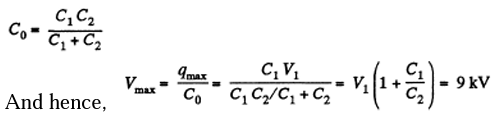15. Find the potential difference between points A and B of the system shown in Figure if the emf is equal E=110 V and the capacitance ratio C2/C1 = η = 2.0.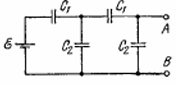Solution:

1. Let us distribute the charges, as shown in the figure.

Now, we know that in a closed circuit, - Δφ = 0

So, in the loop, DCFED,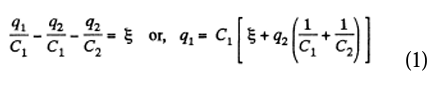Again in the loop DGHED

$\frac{q_{1}}{C_{1}}+\frac{q_{1}+q_{2}}{C_{2}}=\varepsilon$-----(2)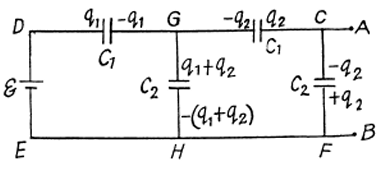Using the above equation we get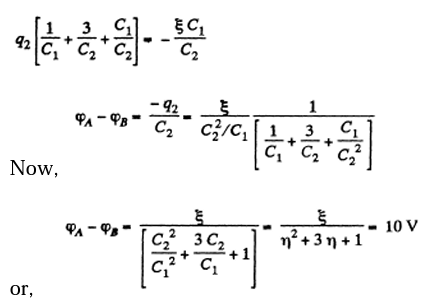16. Find the capacitance of an infinite circuit formed by the repetition of the same link consisting of two identical capacitors, each with capacitance C.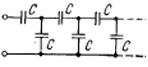Solution:

1. The infinite circuit may be reduced to the circuit, shown in the Figure where C0 is the net capacitance of the combination.

$\frac{1}{C+C_{0}}+\frac{1}{C}=\frac{1}{C_{o}}$

Solving the quadratic equation we get

CCo+ Co2-C2=0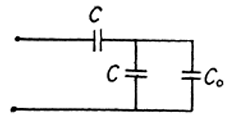$C_{o}=\frac{\sqrt{5}-1}{2}C$

Taking only +ve value as Co cannot be negative.

17. A circuit has a section AB shown in Figure. The emf of the source equalsE=10 V. The capacitor capacitances are equal to C1=1.oμF and C2=2.0 μF and the potential difference ϕAB=5V. Find the voltage across each capacitor.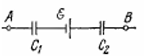Solution:

1. Let, us make the charge distribution, as shown in the figure.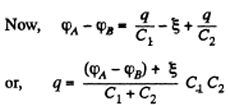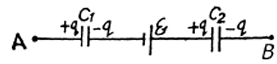Voltage across the capacitors are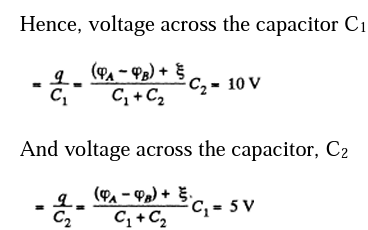18. In a circuit shown in figure. Find the potential difference between the left and right plates of each capacitor.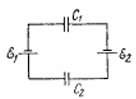Solution:

1. Let $\varepsilon _{2}>\varepsilon _{1}$, then using $-\Delta \phi =0$

We get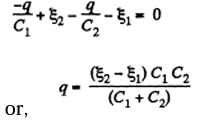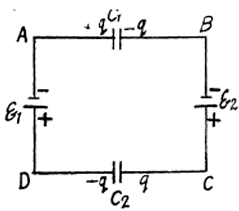Hence the potential difference across the left and right plates of capacitors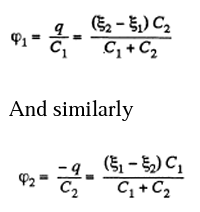19. Find the charge of each capacitor in the circuit shown in the figure.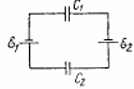Solution:

1. Taking benefit of be foregoing problem, the amount of charge on each capacitor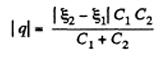20. Determine the potential difference φAφB between points A and B of the circuit shown in the figure. Under what condition is it equal to zero?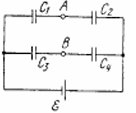Solution:

1. Make the charge distribution, as shown in the figure. In the circuit, In the circuit,12561